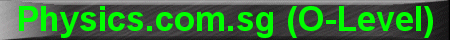(New tips are continually added to these pages.  Check back in a few months' time for more)

TOPIC 14:    Current Electricity

Tip 1:

The equation V = IR causes confusion amongst some students.  When asked what the "V" means, they reply "voltage" or "potential".

"V" is not simply "voltage" or "potential".  "V" is the Potential DIFFERENCE between the two ends of the resistor "R".

In other words, the Potential DIFFERENCE between the two ends of a resistor "R" is numerically equal to the product of its resistance "R" and the current "I" flowing through it.

In the Current Electricity chapter, we do not know, nor do we care, what the absolute potential at each end of the resistor is.  We are only concerned with the DIFFERENCE in potential.

Similarly, we should not confuse the "+" and "-" sign at both ends of the resistor as "Positive" and "Negative".  The "+" simply means that it has a higher potential than the "-" end.

For example,

The "+" end could be at an absolute potential of +12V while the "-" end at +8V (Potential DIFFERENCE = 4V).

Or the "+ end could be at an absolute potential of -5V while the "-" end at -12V (Potential DIFFERENCE = 7V).

Or the "+" end could be at an absolute potential of +3V and the "-" end at -2V (Potential DIFFERENCE = 5V)

Anyway, we are not interested in the absolute potentials.  We are only concerned with the potential DIFFERENCE, which is the "V" in the equation V = IR.